# Queue Notes for GATE

### Introduction to Queue – Study Notes for GATE

The Queue is an important topic that comes under the Computer Science family. And, when we talk about competitive examinations like GATE, you have to delve deeply into every aspect of the Queue. This article will enlighten you with in-depth information about queues. We hope the information provided in the notes for the CSE topics will help you understand this topic in a better way.

What is a Queue?

The Queue is a linear data structure or an abstract data type. Queue follows the FIFO – “first in, first out” method to process the data. The data which is inserted first will be accessed first. Unlike Stack, Queue has two ends REAR and FRONT. The REAR end is always used to insert the data i.e., enqueue, and the FRONT end is used to remove the data inserted i.e. dequeue.

Representation of Queue

Linear array is the best and easiest way to represent the Queue. The Queue contains two ends, REAR and FRONT. These ends point to the position from where the insertion and deletion take place. The REAR points to the variable from where the insertion takes place and the FRONT points to the variable from where deletion takes place.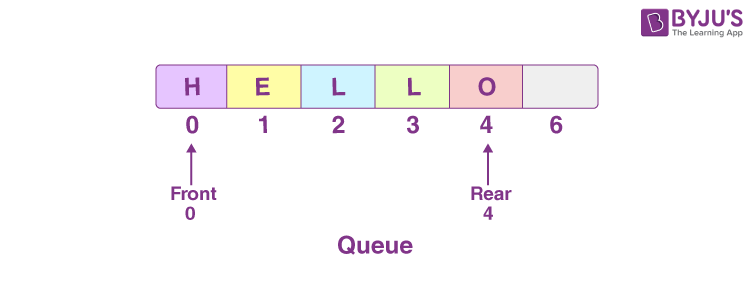Basic Operations in Queue

The enqueue and dequeue are the two basic operations performed in Queue.

Enqueue means to insert any data into the Queue. The steps to insert any data or to enqueue the Queue are as follows:

1. First, we need to check if the Queue is full.
2. If the Queue is full then show the Queue overflow message and exit.
3. If it is not full then the REAR pointer will be incremented to point to the next empty space.
4. Now add the data where the REAR is pointing.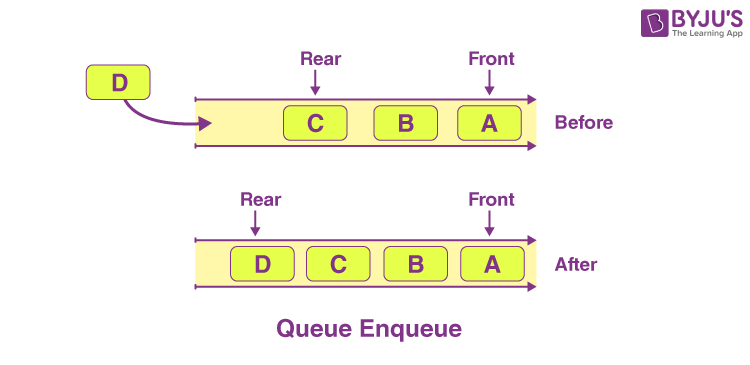Algorithm for Enqueue: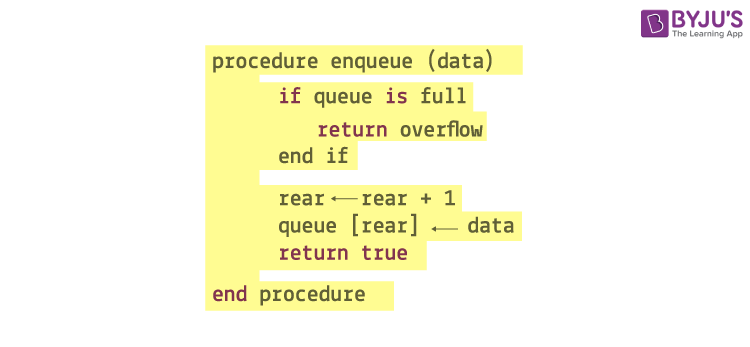Dequeue means to remove any data from Queue. The steps to remove any data from the Queue are as follows:

1. First, you need to check if the Queue is empty.
2. If the Queue is empty, display the Queue underflow message.
3. If the Queue is not empty, then find the data where FRONT is pointing.
4. Now increase the FRONT pointer to point to the available data.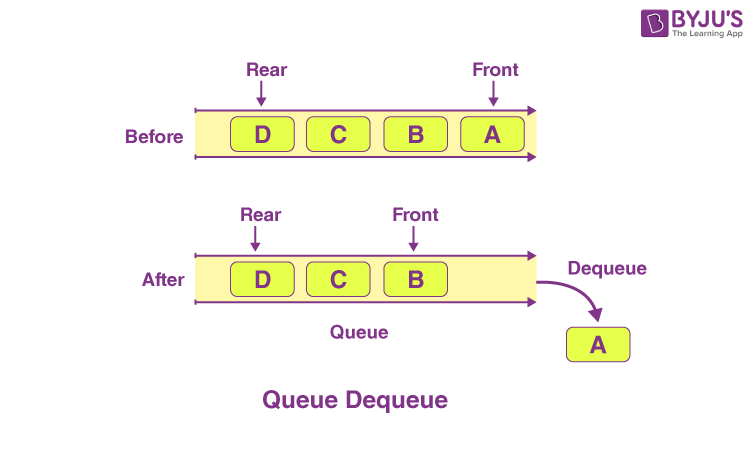Algorithm for Dequeue: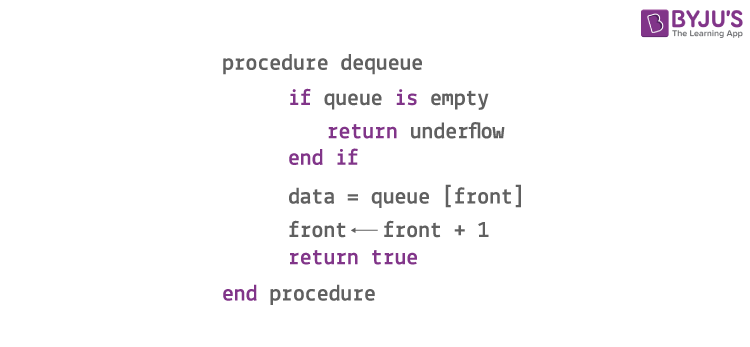Features of Queue:

1. Queue works with FIFO, i.e., First in First Out methodology.
2. Unlike Stack, Queue is also considered as the ordered list of the data that has a similar data type.
3. If any new data is to be inserted in the Queue, then all the data is inserted before it needs to be removed.

## Applications of Queue

When we need to manage any group of data in an ordered manner, where the first inserted data has to be out first, then in such cases Queue needs to be implemented.

1. When we need to serve the request on a single shared device, then the Queue is required. For example a CPU.
2. It removes the conjunction in the processing of the data as it follows FIFO.

### Practice Problem – Queue

Q. A queue is implemented using a non-circular singly linked list. The queue has a head pointer and a tail pointer, as shown in the figure. Let n denote the number of nodes in the queue. Let enqueue be implemented by inserting a new node at the head, and dequeue be implemented by deletion of a node from the tail.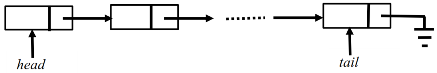Which one of the following is the time complexity of the most time-efficient implementation of enqueue and dequeue, respectively, for this data structure?

• (A) θ(1),θ(1)
• (B) θ(1),θ(n)
• (C) θ(n),θ(1)
• (D) θ(n),θ(n)

## Video on QueueKeep learning and stay tuned to get the latest updates on GATE Exam along with GATE Eligibility CriteriaGATE 2023GATE Admit CardGATE Syllabus for CSE (Computer Science Engineering)GATE CSE NotesGATE CSE Question Paper, and more.

Also Explore,